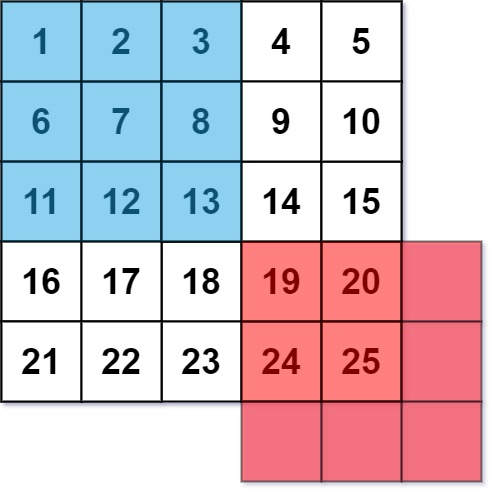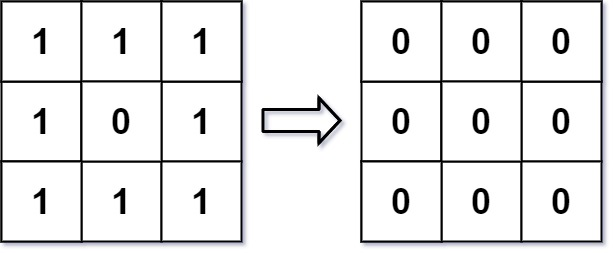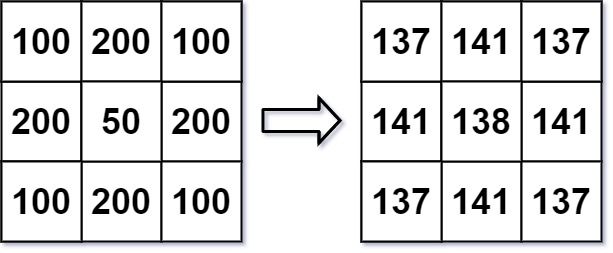661. Image Smoother
Easy
451
1.8K

An image smoother is a filter of the size `3 x 3` that can be applied to each cell of an image by rounding down the average of the cell and the eight surrounding cells (i.e., the average of the nine cells in the blue smoother). If one or more of the surrounding cells of a cell is not present, we do not consider it in the average (i.e., the average of the four cells in the red smoother).Given an `m x n` integer matrix `img` representing the grayscale of an image, return the image after applying the smoother on each cell of it.

Example 1:```Input: img = [[1,1,1],[1,0,1],[1,1,1]]
Output: [[0,0,0],[0,0,0],[0,0,0]]
Explanation:
For the points (0,0), (0,2), (2,0), (2,2): floor(3/4) = floor(0.75) = 0
For the points (0,1), (1,0), (1,2), (2,1): floor(5/6) = floor(0.83333333) = 0
For the point (1,1): floor(8/9) = floor(0.88888889) = 0
```

Example 2:```Input: img = [[100,200,100],[200,50,200],[100,200,100]]
Output: [[137,141,137],[141,138,141],[137,141,137]]
Explanation:
For the points (0,0), (0,2), (2,0), (2,2): floor((100+200+200+50)/4) = floor(137.5) = 137
For the points (0,1), (1,0), (1,2), (2,1): floor((200+200+50+200+100+100)/6) = floor(141.666667) = 141
For the point (1,1): floor((50+200+200+200+200+100+100+100+100)/9) = floor(138.888889) = 138
```

Constraints:

• `m == img.length`
• `n == img[i].length`
• `1 <= m, n <= 200`
• `0 <= img[i][j] <= 255`
Accepted
71.8K
Submissions
129.8K
Acceptance Rate
55.3%

Seen this question in a real interview before?
1/4
Yes
No

Discussion (0)

Related Topics